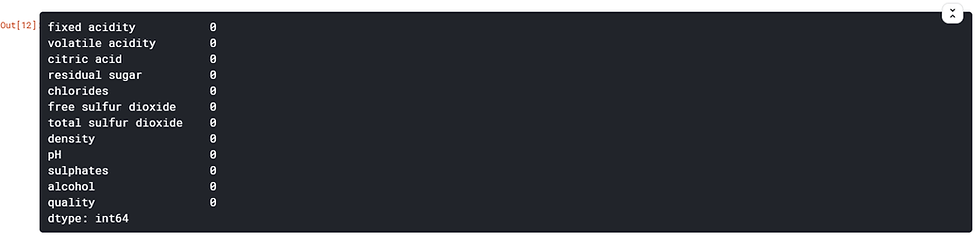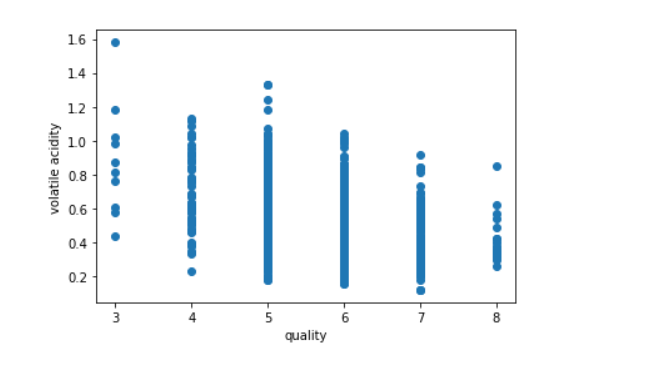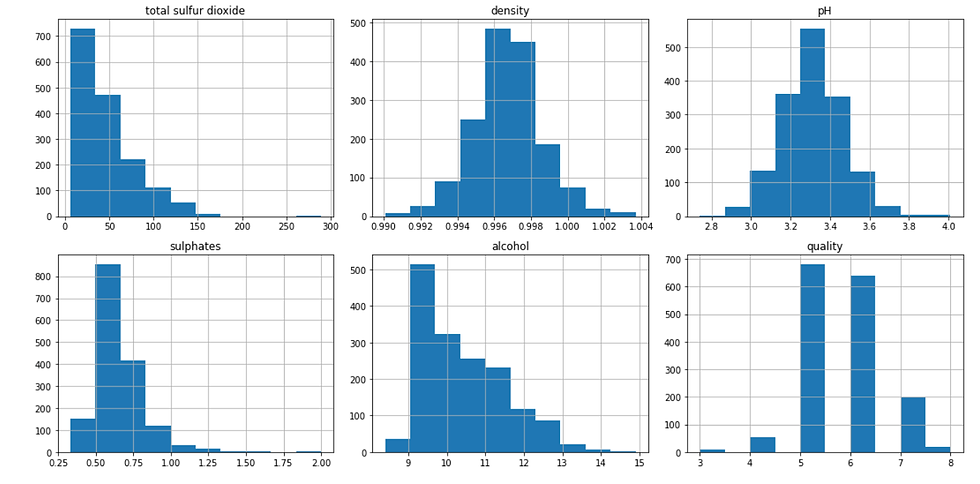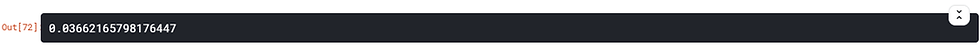top of pageSearch

# Introduction to Exploratory Data Analysis (EDA)Exploratory Data Analysis (EDA) is the process of visualizing and analyzing data to extract insights from it. Sometimes what we see with our naked eye cannot give us all truth. It needs time to understand, analyze and find out the real truth. In other words, EDA is the process of summarizing important characteristics of data in order to gain better understanding of the dataset.

The whole objective of EDA is to understand the data well and understanding the data can be more difficult once we start exploring the data. The EDA is performed to make sure that the data is clean and does not have any redundancies or missing value or null value. We should also identify the most important variable in the data set and remove all the unnecessary noise which may actually hinder the accuracy when building the model.

### Steps involved in EDA

Step 1- Understand the data

Step 2-Clean the data

Step 3- Analyze the relationship between the variables

Step 4- Modelling the data

### EDA explained using sample Data set:

Let me know show you on how EDA works on a data set. Here I am taking Wine Quality data set from Kaggle. To start with I am going to import few important libraries and load data set.

```import pandas as pd
import seaborn as sns
import matplotlib.pyplot as plt
from subprocess import check_output
winequality```Now let’s understand the data

`winequality.head()`The “.head()” function of pandas library by default gives you the top five rows and similarly the “.tail()” function will give you the last five rows.

`winequality.shape `“.shape” command gives you the total number of columns and rows present in the data.

`winequality.info()`“.info()” gives you information about the data. The above data has only float and integer values and no missing value.

`winequality.describe()`This function returns the count, mean, standard deviation, minimum and maximum values and the quantiles of the data. In the above data the mean value is less than the median value of each column. There is also notably large difference between the 75% and max value in few predictors.

`winequality.columns`It gives you various columns name in the data.

`winequality.nunique()`Counts the unique value in the data set.

Now that you have a little bit understanding about the data lets move on to the next step which is cleaning the data.

`winequality.isnull().sum()`This function basically checks for the null value in the data set. There are no null values in this data.

Let’s now move on to the next step which is the visualization of the data and finding the relationship between the variables.

Python has a visualization library, Seaborn which build on top of matplotlib. It provides very attractive statistical graphs in order to perform both Univariate and Multivariate analysis.

In order to use linear regression for the modelling, it is necessary to remove correlated variables to improve the model. We can do that by using pandas “.corr()” and can also the visualize the correlation matrix using heatmap.

`sns.heatmap(correlation, xticklabels=correlation.columns, yticklabels=correlation.columns, annot=True)`The dark shades represent a positive correlation and a lighter shade represents negative. It’s a good to remove correlated variable in feature selection.

Here we can see that “quality” has strong positive correlation with “alcohol” whereas it has strong negative correlation with “volatile acidity”.

Another way of analyzing numerical data would be to use box plots. Box plot shows us the median of the data, which represents where the middle data point is. The upper and lower quartiles represent the 75 and 25 percentiles of the data respectively. The upper and lower extremes show us the extreme ends of the distribution of our data.

```plt.boxplot(winequality)
plt.show()```Lets see the data with scatter plot

A great way to visualize this relationship would be to use a scatter plot. Scatter plots represent each relationship between two continuous variables as individual data point in a 2D graph.

```plt.scatter(winequality['quality'],winequality['volatile acidity'])
plt.xlabel('quality')
plt.ylabel('volatile acidity')
plt.show()```Here I am taking just one variable and checking the graph. We can do this for each of the variable and check the linear relationship between them.

Histogram - Just another way to represent the graph

Histogram shows us the frequency distribution of a variable.

```winequality.hist(figsize=(15,15))
plt.tight_layout()
plt.show()```Let’s build the model now…

```# x= winequality.drop(['quality'], axis=1) choose this columns based on correlations
x= winequality.loc[:,['fixed acidity', 'volatile acidity','residual sugar', 'chlorides', 'total sulfur dioxide',
'pH', 'sulphates', 'alcohol', 'quality']]
y= winequality.loc[:,'quality']
from sklearn.model_selection import train_test_split
xtrain,xtest,ytrain,ytest= train_test_split(x,y,test_size=0.3, random_state=0)```

Finding out accuracy from various models

```from sklearn.linear_model import LinearRegression
lr= LinearRegression()
lr.fit(xtrain,ytrain)
lr.score(xtest,ytest)``````from sklearn.tree import DecisionTreeRegressor
dt= DecisionTreeRegressor(max_depth=5,min_samples_leaf= 20)
dt.fit(xtrain,ytrain)
dt.score(xtest,ytest)``````from sklearn.linear_model import LogisticRegression
l= LogisticRegression()
l.fit(xtrain,ytrain)
l.score(xtest,ytest)``````from sklearn.svm import SVC
svc=SVC()
svc.fit(xtrain,ytrain)
svc.score(xtrain,ytrain)``````from sklearn.model_selection import cross_val_score
cvs= cross_val_score(svc,xtest,ytest,cv=10, scoring='accuracy')
cvs.mean()````cvs.std()````cvs= cross_val_score(rf,xtest,ytest,cv=10, scoring='accuracy')
cvs.mean()```Hope I was able to give you some information on EDA.